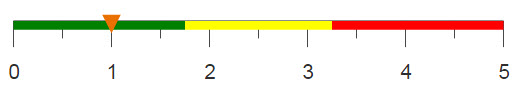# Dividing data into buckets

Dividing data into buckets can make it easier to perform analyses and gather information about the data, especially if the data set is large. If the location of the items are not important, you can randomly divide the data into a predefined number of equal groups.

## Difficulty## Objective

You want to divide your data set evenly into a chosen number of buckets (or groups), where each bucket contains roughly the same number of values. Using characteristics of the data to create the buckets could lead to the groups being uneven. For example, if you’re dividing records on people, using age or gender as a selection basis may lead to unevenly sized groups. In order to ensure that the groups are even, you can randomly distribute the data by using a random number generator.

## Solution

```<base table="pub.doc.retail.altseg.customers"/>
<willbe name="picker" value="draw(12345;10)"/>
<tabu label="Tabulation on Customer Master" breaks="picker">
<tcol source="cust_age" fun="avg" label="Average`age"/>
<tcol source="cust_age" fun="cnt" label="# Records"/>
</tabu>```

## Discussion

To divide your data into an equal number of buckets, you need to create a new column that assigns each record a number to determine their bucket. Say you wanted 10 buckets, then each value in your data set would need to be assigned a number 1 through 10. In order to make these assignments random, you can use the draw(X;Y) function, where the value given to Y is the number of buckets desired.

Additionally, this solution uses tabulations to make sure each bucket created contains roughly the same number of values and that the average of each bucket is comparable.

## Common errors

The query failed after 0.0 seconds with the message: Computed column picker cannot be parsed: Inappropriate argument for "draw"
With the draw(X;Y) function, X is used as a seed, which is used to generate the random numbers before each is assigned a value from Y. This number can be any integer, however if it exceeds 2,147,483,646 you will receive this error.

## Further reading

If you would like to learn more about the functions and operations discussed in this recipe, click on the links below:

draw(X;Y)